Author: Oscar Cronquist Article last updated on January 30, 2019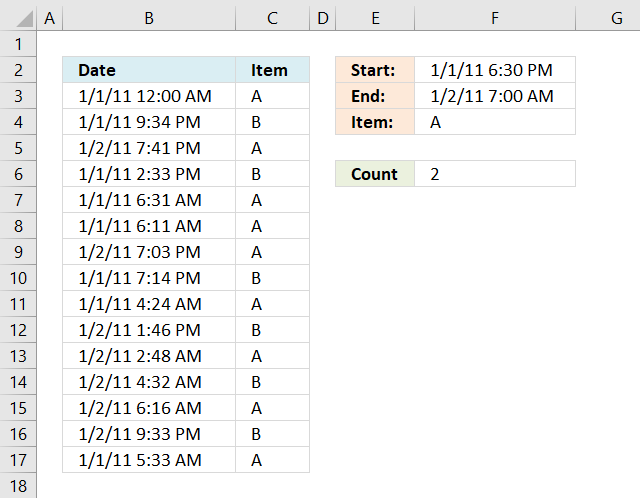Question:

My issue is that I get the date in this format:

7/23/2011 7:00:00 AM

I am trying to count how many entries are between date and time. So I have a shift that starts at 6:30:00 PM and leaves at 7:00:00 AM the next morning. I have tried to convert that to a value to no avail.

I have the cells formatted correctly and tried your other formula =SUM(IF((\$A\$2:\$A\$10\$D\$1),1,0)) + CTRL + SHIFT + ENTER but that returned all records.

I am starting to think that Excel (I'm using 2010) cannot differentiate between the date and time. Any ideas would be greatly appreciated.

Formula in cell F6:

=COUNTIFS(B3:B17, "<="&F3, B3:B17, ">="&F2, C3:C17, F4)

#### Explaining formula in cell F6

The COUNTIFS function was introduced in Excel 2007 and it works like the COUNTIF function except you may use multiple conditions at the same time.

COUNTIFS(criteria_range1, criteria1, [criteria_range2, criteria2]…)

 Pair criteria_range criteria Text 1 B3:B17 "<="&F3 Are dates in B3:B17 smaller than end date in cell F3? 2 B3:B17 ">="&F2 Are dates in B3:B17 larger than start date in cell F2? 3 C3:C17 F4 Are Items in c3:C17 equal to cell F4?

The ampersand character concatenates the logical operators <> and = to each cell or cell range before the COUNTIFS function evaluates the argument. If all conditions return TRUE then the record is counted as 1.

#### Step 1 - Criteria pair 1

The following image shows in column D date and time entries smaller than or equal to condition in cell F3, TRUE - Smaller, FALSE - larger.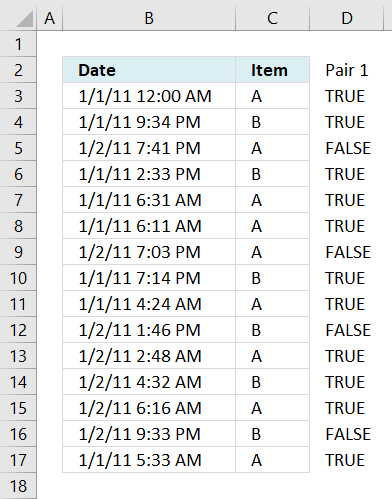#### Step 2 - Criteria pair 2

This image shows in column E date and time entries larger than or equal to condition in cell F2, TRUE - Smaller, FALSE - larger.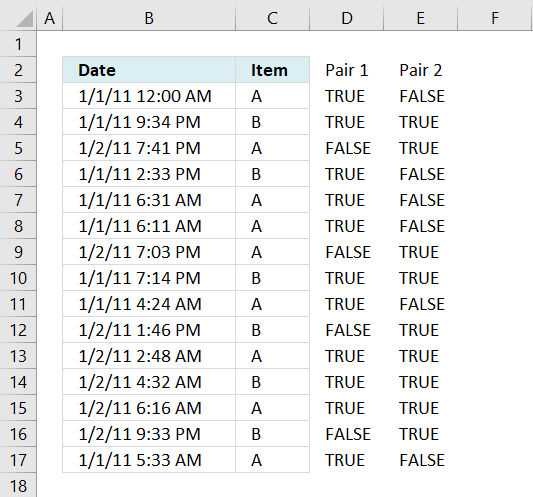#### Step 3 - Criteria pair 3

This picture displays in column F items equal to condition in cell F4.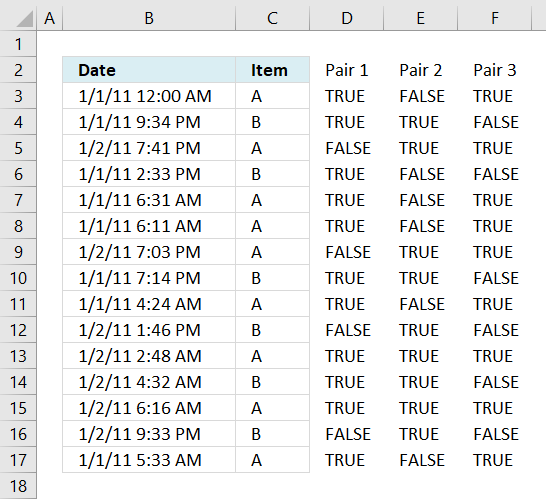#### Step 4 - All conditions applied

This image shows which entries meet all conditions. If all conditions evaluate to TRUE then that specific record is counted, the formula returns 2 in cell F6 because two records meet all conditions.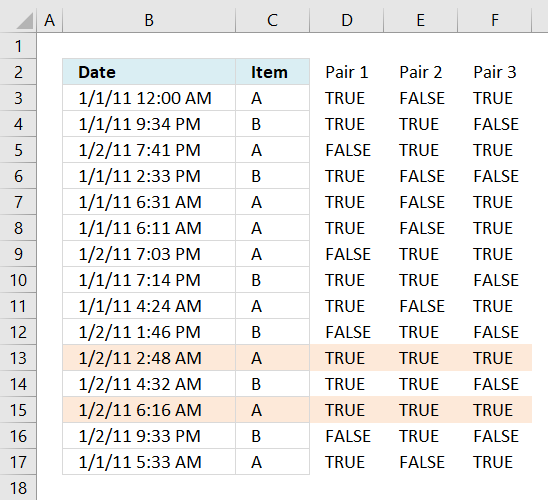Excel 2003 (and earlier versions) formula in cell F6:

=SUMPRODUCT((B3:B17<=F3)*(B3:B17>=F2)*(C3:C17=F4))

### Get the Excel filetoolmanv2.xlsx